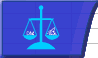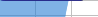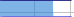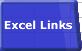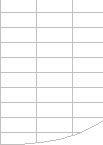# Excel Pages - Optimising SpeedImportance of Speed Faster Calculations Microsoft's Advice Bottlenecks/Size Lookups Dynamic Ranges Totalling Generating Workbooks Multi-Level Calculations Array Formulae SUMIF Example Worksheet LinksBuild Vertically Separate Data Precalculate Constants Replace = Signs Avoid Forward Referencing

 This article mainly applies to versions of Excel that are no longer much used. The Microsoft Knowledge Base has an article called: Q72622 –XL: Optimizing Worksheets for Fastest Calculation I tested the advice given in the article using Excel 97 and Windows 98 on a 300 MHZ Pentium 2 with 128MB RAM. Similar results were obtained using Excel 2000 and Windows 2000. The results and comments are as follows:

### MS Says: Build Vertically: Many rows and few columns are better than many columns and few rows.

Using many columns does not make much difference.

This is no longer true. There is a slight advantage to using as many columns as possible:

#### Horizontal Worksheet:

262143 formulae in 256 columns and 1024 rows

• Filesize:          8190K
• Memory:        12000K
• Calculate:       430 milliseconds @ 300MHZ

#### Vertical Worksheet:

262143 formulae in 4 columns and 65536 rows

• Filesize:          9548K
• Memory:        11900K
• Calculate:       580 milliseconds @ 300MHZ

### MS Says: Keep raw data on a separate sheet.

Putting your data in a separate sheet is a good design rule, but slows down calculation.

This is excellent design advice but poor calculation speed advice: in fact this usually slows down the calculations, and increases memory usage.

#### Referencing Data on the Same Worksheet:

768000 formulae referencing 768000 data cells on the same Worksheet.

• Filesize:          32680K
• Memory:        39090K
• Calculate:       1.3 seconds @ 300MHZ

#### Referencing Data on a Different Worksheet:

768000 formulae referencing 768000 data cells on a different Worksheet.

• Filesize:          32680K
• Memory:        57061K
• Calculate:       4.4 seconds @ 300MHZ

### MS Says: Precalculate Constants.

Good design has simple, easy-to-understand formulae, and all constants stored in separate named cells. Simpler formulae usually calculate faster than complex formulae.

#### Formulae should be as simple as possible: calculate constants before entering them into a formula.

This is good advice as far as it goes from a performance viewpoint, but a good spreadsheet design rule is that formulae should not contain ANY constants: they should be held in separate cells for audit and maintenance reasons.
The best advice is to look carefully at the formulae that are used most often and consume most time to see if they can be simplified, or split into two formulae one of which is executed less frequently.

### MS Says: Activate the Automatic Except Tables Calculation Option.

This is one of Excel’s calculation options.
If you are using Excel’s little-used Table feature this may be sensible.

### MS Says: When you only need a few cells to be recalculated replace the = signs in the formulae.

 This technique is sometimes useful, but make sure that your workbook is correctly calculated. You can use this method to check if you think Excel has failed to calculate a formula. In Manual mode this method calculates only the formulae with replaced = signs, and does not recalculate formulae dependent on the results of this formula. The first time you enter a formula Excel also evaluates it as above.

### MS says: Where possible a formula should refer only to the cells above/before it:

A natural flow of calculation left-to-right and top-to-bottom is excellent design advice,  BUT usually does not affect calculation time.

#### Avoid Forward References.

This is no longer true, at least for simple references, from a calculation time perspective.
It’s still sensible advice because it helps ease of understanding.
Also extreme forward referencing can slow down data entry.

The Forward referencing tests were done using Excel97 and Excel2000 on a single sheet workbook with a continuous chain of formulae, where each formula refers to the cell in before it (Backwards), or after it (Forwards).
Forwards has a chain of formulae running from IV3000 to A1.
Backwards has a chain of formulae running from A1 to IV3000.

There is no significant difference in calculation speed when forward referencing on the same worksheet, although editing can become slow.

#### Forward Referencing:

768000 formulae in 256 columns and 3000 rows

• Filesize:          23786K
• Memory:        33530K
• Calculate: 1.25 seconds @ 300MHZ

#### Backwards Referencing:

768000 formulae in 256 columns and 3000 rows

• Filesize:          23975K
• Memory:        33762K
• Calculate: 1.25 seconds @ 300MHZ
Forward worksheet cross-references  can be very slow.
See FastExcel:
Map CrossReferences .

#### Slow Data Entry with Extreme Forward Referencing:

 In extreme cases forward referencing can slow down data entry. Forward referencing  on the same worksheet will slow down Excel’s rebuilding of the dependency tree . This is not done when calculating, but when the formula is entered or changed. This behavior changed with Excel97. The effect is only noticeable with very deeply nested forward dependencies on large worksheets. If you are in manual calculate mode and notice a pause when you enter a formula this may be the reason. In the Forwards example above changing the number in IV3000 and tabbing to the previous cell whilst in manual calculation mode takes 58 seconds @ 300MHZ. The equivalent for Backwards is nearly instantaneous.© 2001-2020 Decision Models Legal Disclaimer Privacy Statement